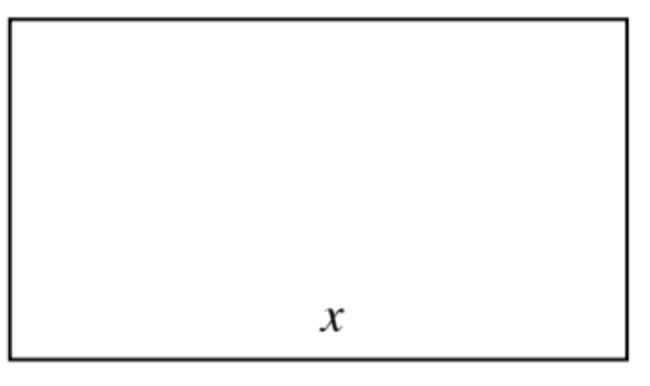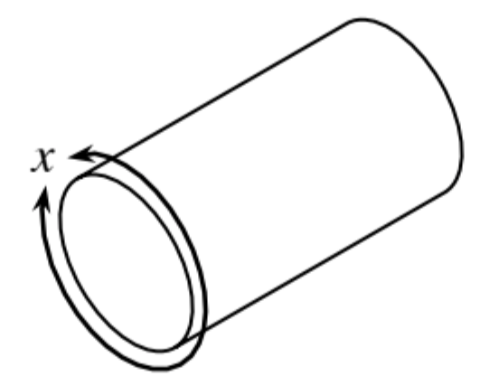### Home > APCALC > Chapter 5 > Lesson 5.1.2 > Problem5-14

5-14.

A rectangular piece of sheet metal with a perimeter of $50$ cm is rolled into a cylinder with two open ends.1. Write equations for the radius and height of the cylinder in terms of $x$.

Look at the diagram of the cylinder. The circumference of the base is equivalent to $x$.

$P = 2b + 2h$
$25 = 2x+2h$
Solve for $h$.

2. Express the volume of the cylinder as a function of $x$.

$V=\pi\left(r^2\right)\left(h\right)$. Substitute.

3. Determine the value of $x$ that will maximize the volume and calculate the maximum value.

The volume will be at a maximum when its derivative is $0$.